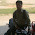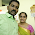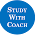Dear Aspirants,Reasoning Questions for IBPS RRB 2018

Reasoning Ability is an onerous section. With the increasing complexity of questions, it becomes hard for one to give it the cold shoulder. The only way to make the grade in this particular section in the forthcoming banking exams like IBPS RRB is to practice continuously with all your heart and soul. And, to let you practice with the best of the latest pattern questions, here is the Adda247 Reasoning Quiz based on the study plan and the exact same pattern of questions that are being asked in the exams.

Direction (1-5): Read the given information carefully and answer the questions;

Six boxes A, B, C, D, E, and F are placed one above another, also they are occupied with different items i.e. O, P, Q, S, N, and M (but not necessarily in the same order).

Box which is occupied with Q is not placed at top. A is placed either top or bottom, only 2 boxes are placed between A and D, which is occupied with S. Only 1 box is placed between D and F. Box E is not placed just above and below D. Box E is not placed just below the box which is occupied with O. Box which is occupied with M is placed between D and F. The box which is placed just above B is occupied with P. The box which is placed at bottom occupied with N. Box B is not placed above A.

Q1. Box C is occupied with which of the following item?
(a) N
(b) P
(c) S
(d) M
(e) None of these

Q2. Which of the following box is placed at top?
(a) A
(b) F
(c) C
(d) E
(e) None of these

Q3. How many boxes are placed between box F and box E?
(a) One
(b) Three
(c) Two
(d) More than three
(e) None of these

Q4. Which box is placed just above box B?
(a) D
(b) The box which is occupied with P
(c) F
(d) Both (b) and (c)
(e) None of these

Q5. Box B is occupied with which of the following item?
(a) N
(b) P
(c) M
(d) Q
(e) None of these

Solutions (1-5):

(i). A is placed either top or bottom, only 2 boxes are placed between A and D, which is occupied with S. The box which is placed bottom occupied with N. Box B is not placed above A. Only 1 box is placed between D and F, so 2 cases formed. Box E is not placed just above and below D. Box which is occupied with M is placed between D and F.

(ii). The box which is placed just above B is occupied with P, so B’s position will be fixed in both cases. Box which is occupied with Q is not placed at top, so B occupied with Q, and C occupied with M in case 1. A occupied with O. Box E is not placed just below the box which is occupied with O, so case 1 will be eliminated and we get final answer.

S1. Ans.(e)
Sol.

S2. Ans.(a)
Sol.

S3. Ans.(b)
Sol.

S4. Ans.(d)
Sol.

S5. Ans.(c)
Sol.

Direction (6-10): Read the given information carefully and answer the questions;

Eight boxes A, B, C, D, E, G, H, and F are placed one above another (but not necessarily in the same order). No two boxes according to alphabetical order are placed adjacent to each other. (A is not placed just above and below B)
Box E is placed at 2nd position either from top or from bottom. Only 2 boxes are between F and C. Box D is placed just below F. Box B is placed above C but not at top. Only 1 box is placed between C and G.

Q6. Which of the following box is placed at top?
(a) C
(b) B
(c) A
(d) H
(e) None of these

Q7. How many boxes are in between box E and box F?
(a) Three
(b) Two
(c) Four
(d) More than four
(e) None of these

Q8. Which of the following box is placed just above box A?
(a) B
(b) H
(c) D
(d) E
(e) None of these

Q9. Which of the following box is placed at bottom?
(a) G
(b) A
(c) B
(d) D
(e) None of these

Q10. Which of the following is true regarding E?
(a) E is placed above A
(b) E is at 2nd position from bottom
(c) C is just below E
(d) E is placed below G
(e) None of these
Solutions (6-10):

(i) No two boxes according to alphabetical order are placed adjacent to each other. Box E is placed at 2nd position either from top or from bottom. Only 2 boxes are between F and C. Box D is placed just below F. Box B is placed above C but not at top. Only 1 box is placed between C and G. by concluding these conditions we will have 2 cases.

(ii) Box B is placed above C but not at top, case1 B will placed at bottom so this case will be cancelled because B is placed above C. In case2 B will be placed at 2nd position from top. A will be placed just above C and H will be paced at top. We get final answer.

S6. Ans.(d)
Sol.

S7. Ans.(a)
Sol.

S8. Ans.(c)
Sol.

S9. Ans.(a)
Sol.

S10. Ans.(b)
Sol.

Direction (11-15): Read the given information carefully and answer the questions;

Seven boxes A, B, C, D, E, G, and F are placed one above another (but not necessarily in the same order) are going to parcel seven different places M, N, O, P, Q, S, and R.

Box which is being parcel to O is just above G. Only three boxes are between G and A. More than four boxes are between the box A and the box which is being parcel to R. Box B is being parcel to N and placed just above box F. Only one box is between F and C. E is not being parcel to R. Only three boxes between the box which is being parcel to M and S cities. The box which is being parcel to S is just above the box which is being parcel to P. E is not being parcel to P.
Box which is being parcel to Q is not F.

Q11. Which of the following box is placed at top?
(a) The box which is being parcel to M
(b) B
(c) C
(d) The box which is being parcel to Q
(e) None of these

Q12. How many boxes are in between box E and box which is being parcel to P?
(a) Three
(b) Two
(c) Four
(d) More than four
(e) None of these

Q13. Which of the following box is placed just above box A?
(a) B
(b) C
(c) D
(d) No one
(e) None of these

Q14. Which of the following box is placed at bottom?
(a) G
(b) A
(c) The box which is being parcel to P
(d) D
(e) None of these

Q15. Which of the following is true regarding E?
(a) E is being parcel to Q
(b) E is at 2nd position from top
(c) C is not just below E
(d) E is placed above G
(e) All are true

Solutions (11-15):

(i). Box which is being parcel to O is just above G. Only three boxes are between G and A. More than four boxes are between the box A and the box which is being parcel to R. Box B is being parcel to N and placed just above box F. Only one box between F and C, by combining these conditions we get 2 cases.

(ii). E is not being parcel to R, so E is placed at 2nd from top in both cases. D placed at top in case 2 and at bottom in case 1. Only three boxes between the box which is being parcel to M and S cities. The box which is being parcel to S is just above the box which is being parcel to P. E is not being parcel to P. Box which is being parcel to Q is not F, by using these conditions case 2 gets eliminated and we get final solution.
S11. Ans.(a)
Sol.

S12. Ans.(a)
Sol.

S13. Ans.(d)
Sol.

S14. Ans.(d)
Sol.

S15. Ans.(e)
Sol.#### 13 comments:

1.2.AMAZING PUZZLES
THANKS ADDA 247

3.This comment has been removed by the author.

4.in third puzzle solution ; this case is a possibility
A-S
C-P
B-N
F-O
G-M
E-Q
D-R

5.in 3rd puzzle there is another possibility as said by sishu

6.same as shishupal sinha

7.It's very useful thanks to bankersadda

8.Given conditions fit but the answers are different..Which one is correct ??

9.Useful puzzles...

10.wrong puzzle cz m or s beach koi ek upor may rahenga aisa condition dene bhul gaya

11.one1 able to solve this kind of puzzle within 3 or 4 mins if yes ,what should be the correct approch

12.Sir to provide puzzle pdf

13.in thirs puzzle 100 per cent is a possiblity...

A-S
C-P
B-N
F-O
G-M
E-Q
D-R